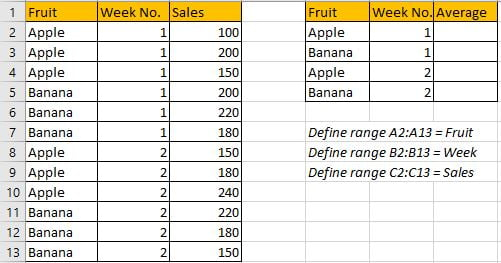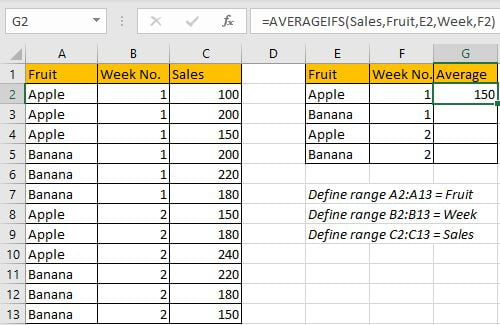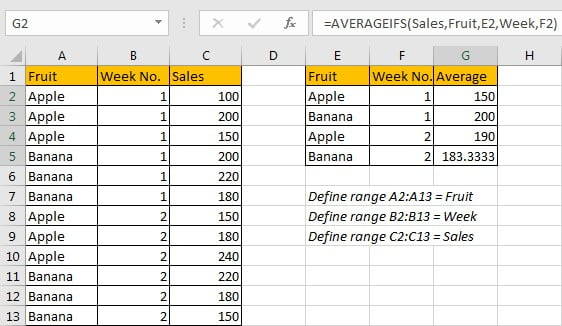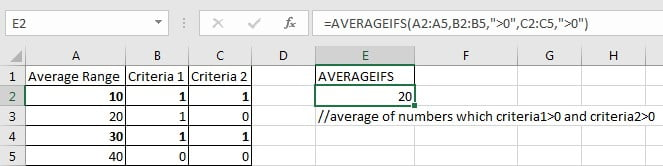# Average with Multiple Criteria in Excel

AVERAGEIFS function is one of the most popular functions in Excel. Apply AVERAGEIFS function, we can calculate average simply if there are multiple conditions.

In this article, we will introduce you to apply AVERAGEIFS function to calculate average of a set of numbers which are filtered by multiple criteria. You can get the basic knowledge and usage of AVERAGEIFS function in this article.

## EXAMPLEIn this case, we want to calculate the average of sales based on different fruit in different week. Obviously, there are two criteria in this case, one is “kind of fruit”, the other one is “week number”. For example, to get average for “apple” in “week1”, we need to look up values from “Sales” which corresponding fruit and week number can satisfy above two conditions.

In this article, to approach our goal, we can apply AVERAGEIFS function.

## SOLUTION

As there are two conditions in this case, so we need to choose a function that can handle multiple conditions, and at the same time can execute average calculation, so AVERAGEIFS is the best choice. If there is only one condition, we can also apply AVERAGEIF function.

## FORMULA with AVERAGEIFS FUNCTION

In G2, we input the formula =AVERAGEIFS(Sales,Fruit,E2,Week,F2). After typing, press Enter, average of sales for different fruit in different weeks is returned properly.Three values in “Sales” can meet our condition “Fruit=Apple & Week Number=1”, there are C2-100, C3-200, C4-150, so the average is (100+200+150)/3=150, the formula returns correct value.

Drag down handle to fill other cells with the same formula in Average column.## FUNCTION INTRODUCTION

In this instance we only apply one function, it is “AVERAGEIFS”, it is one of the most popular functions in Excel. It can be seen as AVERAGE and IFs, similar with SUMIFS, AVERAGIFS can handle multiple criteria when calculating average.

AVERGAEIFS function returns the average of a set of numbers in range based on one or more given conditions or criteria.

Syntax:

`=AVERAGEIFS (average_range, criteria_range1, criteria1, [criteria_range2], [criteria2], ...)`

For AVERAGEIFS functions, it can handle wildcards like asterisk ‘*’ and question mark ‘?’; it also supports logical operations like ‘>=’,’<=’. If we need entering wildcards or logical operators to build criteria, they should be enclosed into double quotes (““).

Example.## FORMULA EXPLANATION

`=AVERAGEIFS(Sales,Fruit,E2,Week,F2)`

// average_range is named range “Sales”.

//criteria_range1 is named range “Fruit”, criteria1 is value in E2.

//criteria_range2 is named range “Week”, criteria2 is value in F2.

a. For the first group of criteria range and criteria, expand values in “Fruit” and “E2”:

`{"Apple";"Apple";"Apple";"Banana";"Banana";"Banana";"Apple";"Apple";"Apple";"Banana";"Banana";"Banana"},"Apple"`

Filter data from “Fruit” refer to criteria1 “Fruit=Apple”:

`{TRUE;TRUE;TRUE;FALSE;FALSE;FALSE;TRUE;TRUE;TRUE;FALSE;FALSE;FALSE}`

b. For the second group of criteria range and criteria, expand values in “Week” and “F2”:

`{1;1;1;1;1;1;2;2;2;2;2;2},1`

Filter data from “Week” refer to criteria2 “Week=1”:

`{TRUE;TRUE;TRUE;TRUE;TRUE;TRUE;FALSE;FALSE;FALSE;FALSE;FALSE;FALSE}`

c. After filtering data from two groups, we can notice that only the first three positions in both two arrays are “TRUE”. Thus we only need to extract the first three values from average range and then come to AVERAGE calculation.

`{100;200;150}`

After above all steps, we get =AVERAGE({100;200;150}). So, the returned value is (100+200+150)/3=150.

### Related Functions

• Excel AVERAGE function
The Excel AVERAGE function returns the average of the numbers that you provided.The syntax of the AVERAGE function is as below:=AVERAGE (number1,[number2],…)….
• Excel AVERAGEIF function
The Excel AVERAGEAIF function returns the average of all numbers in a range of cells that meet a given criteria.The syntax of the AVERAGEIF function is as below:= AVERAGEIF (range, criteria, [average_range])….
• Excel AVERAGEIFS function
The Excel AVERAGEAIFS function returns the average of all numbers in a range of cells that meet multiple criteria.The syntax of the AVERAGEIFS function is as below:= AVERAGEIFS (average_range, criteria_range1, criteria1, [criteria_range2, criteria2],…)….